# JP3770737B2 - Imaging device - Google Patents

## Info

Publication number
JP3770737B2
JP3770737B2 JP29219898A JP29219898A JP3770737B2 JP 3770737 B2 JP3770737 B2 JP 3770737B2 JP 29219898 A JP29219898 A JP 29219898A JP 29219898 A JP29219898 A JP 29219898A JP 3770737 B2 JP3770737 B2 JP 3770737B2
Authority
JP
Japan
Prior art keywords
signal
image
color
means
correlation
Prior art date
Legal status (The legal status is an assumption and is not a legal conclusion. Google has not performed a legal analysis and makes no representation as to the accuracy of the status listed.)
Expired - Fee Related
Application number
JP29219898A
Other languages
Japanese (ja)
Other versions
JP2000125169A (en
Inventor

Original Assignee

Priority date (The priority date is an assumption and is not a legal conclusion. Google has not performed a legal analysis and makes no representation as to the accuracy of the date listed.)
Filing date
Publication date
Application filed by 三菱電機株式会社 filed Critical 三菱電機株式会社
Priority to JP29219898A priority Critical patent/JP3770737B2/en
Publication of JP2000125169A publication Critical patent/JP2000125169A/en
Application granted granted Critical
Publication of JP3770737B2 publication Critical patent/JP3770737B2/en
Anticipated expiration legal-status Critical
Application status is Expired - Fee Related legal-status Critical

## Images

•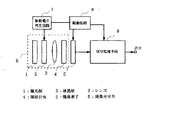•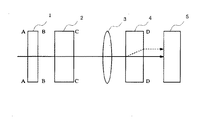••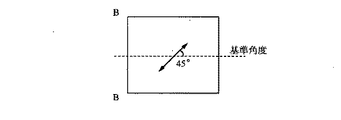•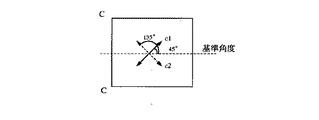•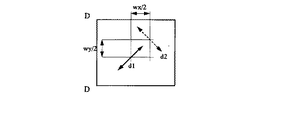••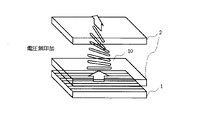••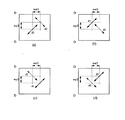•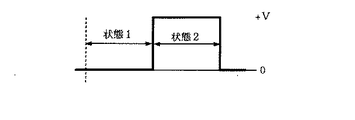•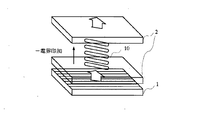•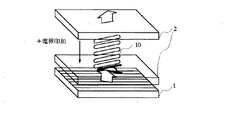•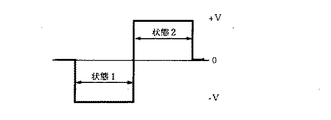•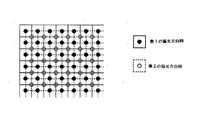•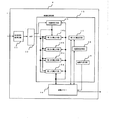•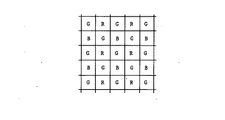•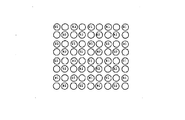•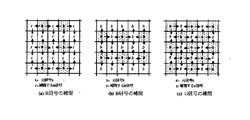•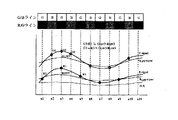•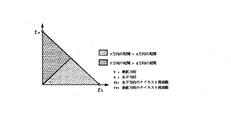•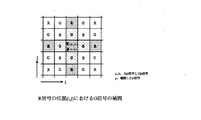•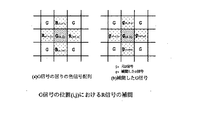•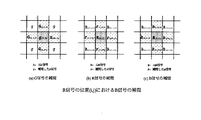•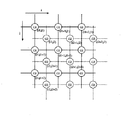•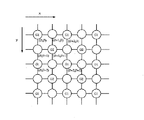•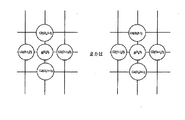•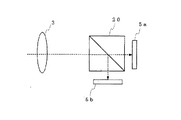••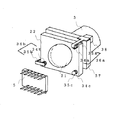•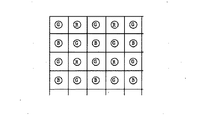•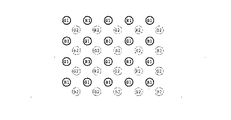•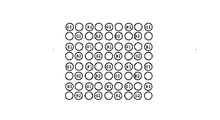••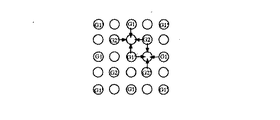•••## Description


BACKGROUND OF THE INVENTION
The present invention provides a high-capacity image signal that obtains a high-definition image signal by shifting pixels in a single-plate color imaging device such as a digital still camera or a digital camera that obtains a color image signal using only one image sensor. It relates to resolution technology.

[Prior art]
In an image input device using an image sensor in which a plurality of pixels such as a CCD are arranged two-dimensionally, each image signal is input after the image signal is input every time the relative position between the image signal and the image sensor is changed and changed. Is known to improve the resolution by increasing the apparent number of pixels.
In order to improve the resolution by such a method, a conventional technique is well known such as a three-plate system in which light is divided into RGB colors by a prism and images are formed on three image sensors.
On the other hand, in an imaging method using two imaging elements in which a plurality of color filters are arranged on one imaging element, an image from the imaging optical system 3 is converted into an optical element such as a half mirror as shown in FIG. 20 forms an image on the two image sensors 5a and 5b.
Each of the image sensors 5a and 5b is arranged so that an image signal formed spatially is imaged with a shift of 1/2 pixel.

In addition, when pixel shifting is performed with only one image sensor, a flat plate transparent member is arranged in parallel between the image sensor and the image sensor as described in, for example, Japanese Patent Application Laid-Open No. 7-236086. The incident light from the photographic optical system is displaced by using one of the three tilting means not on the straight line arranged on the transparent member as a support portion and the other two points as an operation portion for operating the transparent member. In some cases, the image signal on the image sensor is slightly displaced.

FIG. 29 is a block diagram of a conventional image input device for optically increasing the number of pixels disclosed in Japanese Patent Laid-Open No. 7-236086, and FIG. 30 is a diagram showing a mechanism of the imaging unit.
29 and 30, 3 is a lens system for forming an image, 5 is a two-dimensionally arranged image sensor such as a CCD for photoelectrically converting the image, and 21 is a lens system 3 and the image sensor 5. A transparent flat plate member disposed substantially in parallel between the lens system 3 and causing a slight displacement in the incident angle of the incident light from the lens system 3 to the image pickup device 5, and 22 is a base unit for supporting the lens system 3 and the transparent flat plate member 21, and 34a and 34b. , 34c are compression springs for fixing the transparent flat plate member 21 to the base unit 22 and selectively operating two points to incline the transparent flat plate unit.

35a, 35b, and 35c are spring pressing plates that respectively hold the corresponding compression springs 34, and 38a and 38b (not shown) are provided with screws that pass through the base unit 22 and the compression springs 34a and 34b, and are driven by a transparent flat plate. Motors 36a and 36b that cause the transparent flat plate member 21 to be inclined by displacing the vicinity of the member 21 in the optical axis direction. First and second including the transparent flat plate member 21, the compression springs 34a and 34b, and the spring pressing plates 35a and 35b. It is an operating part.
Reference numeral 37 denotes a support portion including the transparent flat plate member 21, the compression spring 34c, and the spring pressing plate 35c. The transparent flat plate member 21 is supported.
These are integrally fixed to a housing (not shown) and, as shown in FIG. 29, a predetermined stage comprising a color signal separation circuit 25, a process circuit 26, etc. for processing a photoelectrically converted image signal signal as shown in FIG. The image signal processing circuit, the image signal synthesis memory 30, the image buffer memory 29, and the like are connected.

Next, the operation will be described.
First, imaging is performed in a state where neither the spring pressing plate 35a of the first operating portion 36a or the spring pressing plate 35b of the second operating portion 36b is operated, and an image signal is stored in the image buffer memory 29 in the subsequent stage.
Next, when one of the two spring pressing plates 35a is operated, the transparent flat plate member 21 rotates with the line connecting the other spring pressing plate 35b and the support portion as the rotation axis.
Therefore, the image transmitted through the transparent flat plate member 21 is moved by the inclination of the transparent flat plate member 21 to form an image on the image sensor 5, and the image signal slightly shifted is stored in the image buffer memory 29.

Further, if the same single spring pressing plate 35a is operated, images are sequentially moved in the same direction, and images are sequentially formed and stored on the image sensor 5.
Further, when the spring pressing plate 35b is driven, the transparent flat plate member 21 is inclined with a line connecting the spring pressing plate 35a and the support portion 37 as a rotation axis, and the image is moved in a direction different from the above.
By appropriately combining these two-direction movements, two-dimensional pixel shifting to an arbitrary position is performed, and each of the plurality of captured images stored in the image buffer memory 29 is considered in consideration of the pixel shifting execution direction. An image signal in which the number of pixels is optically increased by interpolation for each pixel is obtained in the image synthesis memory 30.

Next, a conventional signal processing method for generating a high-definition image from an image signal obtained by conventional pixel shifting will be described.
The signal array shown in FIG. 31 is an image sensor having a Bayer array that is most often used in a single-plate image storage. The signal indicated by a circle in the figure is sampled at the pixel position on the arrangement of the image sensor. It is a color signal.
FIG. 32 is a diagram in which the color signal of the image signal captured by shifting the image sensor shown in FIG.
In the figure, R1, G1, and B1 indicated by solid lines are the color signals of the previous image, and R2, G2, and B3 indicated by hatching are the color signals obtained by pixel shifting.

A color signal array for improving the resolution from the two image signals with the pixel shift shown in FIG. 32 is as shown in FIG.
The number of pixels of the image signal shown in FIG. 33 is twice as large as that in FIG.
However, since the color signals obtained by actually shifting the pixels as shown in FIG. 33 are R1, R2, G1, G2, B1, and B2, the blank color signals in the figure are interpolated from the front, rear, left, and right pixels. There is a need to.

For example, if only the G signal is focused now, there are G signals G1 and G2 obtained by imaging only at the positions shown in FIG.
In the conventional technique, G1 ′ and G2 ′ are interpolated from the average values of the front, rear, left and right signals, and then further interpolated from the G signals obtained by interpolation as shown in FIG. Can be obtained.

Focusing on the B signal, as shown in FIG. 36, the B1 ′ signal is interpolated from the left and right B1 signals, and further the B1 ″ signal is interpolated from the interpolated B1 ′ signal. The same applies to the B2 signal.
Next, as shown in FIG. 37, interpolation of the remaining pixels is performed from the interpolated B1 ′, B1 ″, B2 ′, B2 ″. Also for the R signal, signals for all pixels can be interpolated in the same manner as for the B signal. By the above method, R, G, B signals for all pixels can be obtained.

[Problems to be solved by the invention]
However, the conventional technology as shown in FIG. 28 requires a plurality of image sensors, and it is necessary to dispose each image sensor spatially by ½ pixel while reducing the cost of the image sensor. Now that small image sensors are prosperous, each pixel constituting the image sensor is very fine, so it is very difficult to dispose the image sensor by shifting by 1/2 pixel. Needed a measuring instrument.

In the invention disclosed in Japanese Patent Laid-Open No. 7-236086, the transparent flat plate member is mechanically driven and controlled by two motors, so that it is difficult to realize a high pixel shift amount.
In particular, in recent years, the pixel pitch is about several microns, and a complicated control system is required to obtain a mechanical accuracy that is a fraction of that. In addition, since control is performed using mechanical vibration, a design that fully considers vibration and repeated life is required.

Furthermore, in conventional signal processing for generating a high-resolution image from an image signal imaged by the above method, since it is simple linear interpolation, it is not possible to obtain a resolution corresponding to the number of pixels obtained by performing pixel shifting.
First, since the image signal in FIG. 30 in the single-plate image sensor has a color filter of one color disposed on each pixel, R, G, and B signals corresponding to the number of pixels cannot be obtained, only the number of pixels. The first cause is that the resolution is not obtained, and there is a problem that the linear interpolation cannot sufficiently improve the resolution when the high resolution image is obtained from the two image signals.

Furthermore, when the pixel lacking the signal is interpolated by the above method, the signal arrangement of the image signal for the interpolation is not evenly arranged as shown in FIG. The signal arrangement is modulated in the direction, and if the signal is interpolated as it is, there is a problem that the false color becomes conspicuous.
In order to solve this problem, if the G signal in one image signal is G1, as shown in FIG. 38, four image signals G2, G3, and G4 having undergone pixel shifting are captured and each image signal is taken. Since a high-definition image has to be generated from this, it takes a long time to capture images, and there is a problem that the amount of memory for temporarily storing and holding those image signals in order to generate a high-definition image increases.

The present invention has been made to solve the above-described problems, eliminates the need for a mechanical pixel shifting mechanism that mechanically vibrates the imaging optical system, and further uses only one imaging element in both the horizontal and vertical directions. An object of the present invention is to obtain a highly reliable image pickup apparatus that can switch and output two image signal signals shifted by ½ pixel.
In addition, in each of two image signals shifted by ½ pixel output from the image sensor, an optimal color signal is interpolated adaptively with respect to the pixel position where the signal is missing, so that horizontal / vertical It is an object of the present invention to obtain an imaging device capable of generating two high-resolution image signals that are shifted by 1/2 pixel in each direction.
It is another object of the present invention to obtain an imaging apparatus capable of obtaining a high-definition image by using the above-described two high-resolution image signals shifted by ½ pixel in both the horizontal and vertical directions.

[Means for Solving the Problems]
The image pickup apparatus according to the present invention guides reflected light from an object to be picked up to an image pickup element composed of a plurality of pixels arranged two-dimensionally by an image pickup optical system, and photoelectrically converts the image signal by the image pickup element. In the image pickup apparatus to be generated, the image pickup optical system converts the reflected light from the object to be picked up into a linearly polarized light beam having a first polarization direction with respect to the horizontal direction of the pixel array of the image pickup element and emits the straight line. Corresponding to ON or OFF of the polarization conversion means and the applied voltage, the linearly polarized light beam from the linear polarization conversion means is the first polarized light beam having the first polarization direction or the first polarization direction. A polarization direction switching means for switching and emitting a second polarized light beam having a second polarization direction orthogonal to the first polarization direction, the polarization direction switching means and the imaging device, and the first direction from the polarization direction switching means Polarized light Is obtained by a polarization beam shifting means emits shifted by 1/2 in the horizontal direction and each inter-pixel distance in the vertical direction of the pixel array of the imaging element the second polarized light for.

The polarization direction switching means of the image pickup apparatus according to the present invention is a liquid crystal plate.

The voltage applied to the liquid crystal plate, which is the polarization direction switching means of the imaging apparatus according to the present invention, is not applied at the same time as the imaging device finishes imaging.

The image pickup device of the image pickup apparatus according to the present invention uses the upper and lower four pixels in the vertical two rows and two horizontal columns in the plurality of pixels arranged two-dimensionally in the horizontal direction and the vertical direction as the unit area. A first color filter having spectral sensitivity characteristics with respect to a signal is arranged in the first row and first column and the second row and second column, and a second color filter having spectral sensitivity characteristics with respect to the second color signal is provided in the first row and second column. A third color filter having spectral sensitivity characteristics with respect to the third color signal is arranged in the second row and the first column, and the color filters of the upper and lower four pixels are sequentially arranged in the vertical and horizontal directions, and First correlation determining means for determining a horizontal and vertical correlation at a predetermined pixel position based on a peripheral pixel signal at a predetermined pixel position of the first color signal by the first color filter; In the determination result of the correlation determination means Then, the first color signals at the second and third color signal positions are adaptively applied by the first, second and third color signals read out by the first, second and third color filters. Based on the determination result of the first calculation means for calculating and the first correlation determination means, the output of the first calculation means and the first, second, and third color filters read by the first color filter. Second calculation means for adaptively calculating the second and third color signals at the first color signal position based on the first, second, and third color signals, and a determination result of the first correlation determination means; Based on the output of the first calculation means, the output of the second calculation means, and the first, second, and third colors read by the first, second, and third color filters. A third calculating means for adaptively calculating a third color signal at the second color signal position by the signal; The second color at the third color signal position by the first, second and third color signals read out by the first, second and third color filters based on the determination result of the correlation determination means A fourth calculation means for adaptively calculating a signal, and a first image signal obtained in the first polarization direction and a second image signal obtained in the second polarization direction, The color signals calculated by the first to fourth calculation means are used to interpolate the first, second, and third color signals at pixel positions where signals are missing.

Further, the first correlation determination unit of the imaging device according to the present invention calculates an absolute value of a difference between left and right adjacent pixels at a predetermined pixel position of the first color signal, and detects a horizontal edge component. Edge detection means; vertical edge detection means for detecting an edge component in the vertical direction by calculating an absolute value of a difference between upper and lower pixels at a predetermined pixel position of the first color signal; and By comparing the output magnitudes of the direction edge detection means, it is determined whether the correlation between the horizontal and vertical directions in the predetermined pixel is high, and the horizontal edge detection means and the vertical edge detection means And determining means for determining that the correlation is high in both the horizontal direction and the vertical direction when both outputs are smaller than a predetermined value.

The image pickup apparatus according to the present invention is obtained when the first image signal on the image pickup element obtained in the first polarization direction and in the second polarization direction, and the first image signal is horizontal. And an image pickup apparatus that generates a third image signal from a second image signal that is shifted by a half of the inter-pixel distance in the vertical direction, and the position (i, j) in the first image signal (i, j) j is a natural number), the first to third color signals at the position (2i-1, 2j-1) in the third image signal, and the second image signal. Image signal generating means for converting the first to third color signals at the position (i, j) in the first to third color signals at the position (2i, 2j) in the third image signal; 3 at the predetermined pixel position (2i-1, 2j) or (2i, 2j-1) in the image signal 3 The first to third color signals at the predetermined pixel position are adaptively applied based on the determination result of the second correlation determination unit for determining the correlation at the predetermined pixel position and the determination result of the second correlation determination unit And a fifth calculating means for calculating the first to third color signals of all the pixels in the third image signal.

In addition, the second correlation determination unit of the imaging apparatus according to the present invention is configured to calculate a difference between left and right adjacent pixels at a predetermined pixel position (2i-1, 2j) or (2i, 2j-1) in the third image signal. Horizontal edge detection means for detecting an edge component in the horizontal direction by calculating an absolute value and vertical edge detection for detecting an edge component in the vertical direction by calculating an absolute value of a difference between upper and lower pixels at the predetermined pixel position And the horizontal direction edge detecting means and the vertical direction edge detecting means are compared to determine whether the correlation between the horizontal direction and the vertical direction of the predetermined pixel is high. Determination means for determining that the correlation between the horizontal direction and the vertical direction is high when both the output of the direction edge detection means and the vertical direction edge detection means are smaller than a predetermined value; It includes those were.

DETAILED DESCRIPTION OF THE INVENTION
An embodiment of the present invention will be described with reference to the drawings. In the figure, the same reference numerals as in the prior art represent the same or equivalent ones in the prior art.
Embodiment 1 FIG.
FIG. 1 is a diagram showing the overall configuration of an imaging apparatus according to Embodiment 1 of the present invention, and FIG. 2 is an enlarged view for explaining the operation of the imaging optical system in FIG.
1 and 2, reference numeral 1 denotes a polarizing plate that converts incident light into linearly polarized light, 2 denotes a liquid crystal plate, 3 denotes a lens system, 4 denotes a birefringent plate having a refractive index different depending on the polarization direction of the incident light, and 5 denotes a plurality of pixels. 6 is an imaging optical system that generically refers to the polarizing plate 1 to the birefringent plate 4, 7 is a drive voltage generating circuit that applies a drive voltage to the liquid crystal plate, and 8 is for driving the image sensor 5. Numeral 9 is a signal processing circuit for processing the video signal obtained from the image sensor 5.

The operation of the imaging apparatus configured as described above will be described. An enlarged view of the imaging optical system 6 and the imaging element 5 is shown in FIG.
In FIG. 2, AA is the incident side of the polarizing plate 1, BB is the outgoing side of the polarizing plate 1, CC is the outgoing side of the liquid crystal plate, and DD is the vertical direction on the outgoing side of the birefringent plate 4. Indicates the position.
3 to 6 show the polarization directions of light at the respective positions from AA to DD shown in FIG.

When the subject is imaged, the light incident on the imaging optical system 6 (that is, the reflected light from the object to be imaged) is non-polarized light, so that the vertical polarization component and the horizontal polarization component as shown in FIG. Can be shown.
The polarizing plate 1 emits light linearly polarized from the incident light. Further, as shown in FIG. 4, the azimuth angle of the polarizing plate 1 is the polarization axis in the polarization direction so that the azimuth angle is 45 ° with respect to the reference angle when the horizontal pixel array of the image sensor 5 is the reference angle. Tilt to place. That is, the polarizing plate 1 has a function as linearly polarized light conversion means.

The liquid crystal plate 2 has a liquid crystal phase inside and sandwiches the liquid crystal between two electrode substrates. When a voltage, that is, electrolysis is applied from the outside, an electro-optic effect associated with a change in the molecular arrangement of the internal liquid crystal is caused. .
The liquid crystal plate 2 used in the present embodiment is a thing that changes the polarization direction of incident linearly polarized light by turning on or off an applied voltage (drive voltage).
As an example for realizing the liquid crystal plate 2, an optical rotation effect by a twisted nematic (TN) type liquid crystal, which is a typical electrolytic effect type among the electro-optical effects, can be cited. The operation is shown in FIGS.

The nematic liquid crystal exhibiting the TN mode has positive dielectric anisotropy, and the molecular arrangement of the element is shown in FIGS.
7 and 8, 10 is a liquid crystal molecule. When a voltage is applied to the substrate, as shown in FIG. 7, the molecular long axis direction is arranged so as to be perpendicular to both substrate surfaces, so that the linearly polarized light incident through the polarizing plate 1 is emitted as it is. .
When no voltage is applied, the alignment of the liquid crystal molecules in the device is continuously twisted by 90 °, and optically causes a 90 ° optical rotation effect. Therefore, the linearly polarized light incident from the polarizing plate 1 is twisted by 90 °. The outgoing light has a 90 ° polarization direction different from that of the incident light.
That is, the liquid crystal plate 2 has a function of polarization direction switching means.

The polarization of the light emitted from the liquid crystal plate 2 is shown in FIG.
In FIG. 5, c1 is a state in which a voltage is applied to the liquid crystal plate 2, and when no voltage is applied, linearly polarized light having an azimuth angle of 135 ° with respect to the reference angle is obtained, as indicated by c2 in FIG.
Now, let C1 linear polarization be the first polarization direction, and c2 linear polarization be the second polarization direction.
The light emitted from the liquid crystal plate 2 then enters the birefringent plate 4.

The birefringent plate 4 is a material having a so-called birefringence in which the refractive index is not uniform depending on the polarization direction. In the imaging apparatus, when the spatial frequency of the subject exceeds the sampling frequency obtained from the pixel pitch of the imaging element 5, a repetitive noise is generated. Since it appears in the image as (aliasing noise), a quartz plate or the like is often used as an optical low-pass filter.
The quartz plate, that is, the birefringent plate 4 separates incident light into ordinary rays and extraordinary rays, and the separation distance can be adjusted by the thickness of the birefringent plate 4.

When the birefringent plate 4 is provided so that the incident light in the first polarization direction is an ordinary ray and the incident light in the second polarization direction is an extraordinary ray, the extraordinary ray is indicated by a dotted line as shown in FIG. The optical axis is different from that of ordinary light, and incident light having the respective polarization directions forms images at different positions on the image sensor 5 as indicated by d1 and d2 in FIG.
When the birefringent plate 4 is normally used as the optical low-pass filter (LPF), the thickness of the birefringent plate 4 is such that the crystal is notched in the horizontal direction in order to separate the ordinary ray and the above ray, and in the vertical direction. A birefringent plate is formed by stacking a plurality of crystal cutouts in the direction that separates the ordinary light beam and the above light beam. In the imaging optical system of this imaging device, the pixel pitch in the horizontal direction of the imaging device The direction of separating the birefringent plate 4 having a thickness for separating the ordinary ray and the above ray by (Wx ↑ 2 + Wy ↑ 2) ↑ (1/2) with respect to Wx and the pixel pitch Wy in the vertical direction is imaged. It is installed at an angle of 45 degrees with respect to the element.

Since the first polarization direction is 45 degrees and the second polarization direction is 135 degrees, the light beam that has passed through the birefringent plate 4 provided as described above has a horizontal direction as shown in FIG. The extraordinary ray differs from the ordinary ray by Wx / 2 which is half of the pixel pitch Wx of the image sensor and Wy / 2 which is half of the pixel pitch Wy of the image sensor in the vertical direction, and is imaged on the image sensor 5. Is done. In this way, the polarization axis of the polarizing plate is inclined 45 degrees with respect to the image sensor, and the separation direction of the birefringent plate is set to 45 degrees, so that the birefringent plate 4 made of a single crystal or the like is used at a time. It is possible to shift pixels by 1/2 pixel in the horizontal direction and 1/2 pixel in the vertical direction.
That is, the birefringent plate 4 shifts the polarized light beam having the first and second polarization directions orthogonal to each other from the crystal plate 2 by 1/2 pixel in the horizontal direction and 1/2 pixel in the vertical direction. It has the function of a polarized light beam shifting means.

Therefore, the two image signals of the first image signal obtained by imaging the image formed on the image sensor 5 in the first polarization direction and the second image signal imaged in the second polarization direction are: The horizontal and vertical directions are relatively displaced by 1/2 pixel.
From the above, the image pickup apparatus outputs a signal to the drive voltage generation circuit 7 when the image pickup device 5 is driven by the drive circuit 8, and the drive voltage generation circuit 7 outputs the drive voltage to the liquid crystal plate 3. When the voltage is applied to the liquid crystal plate 2, the liquid crystal molecular arrangement shown in FIG. 7 is formed, and incident light in the first polarization direction is imaged on the image sensor 5. The image sensor 5 captures an image with a drive signal from the drive circuit 8 to obtain a first image signal.

Next, after imaging the first image signal, the drive circuit 8 outputs a signal to the drive voltage generation circuit 7, and the drive voltage output circuit 7 sets the applied voltage to 0 on the liquid crystal plate 2. The liquid crystal molecular arrangement of the liquid crystal plate 2 is as shown in FIG. 8, and incident light in the second polarization direction is imaged on the image sensor 5. The image sensor 5 captures an image with a drive signal from the drive circuit 8 to obtain a second image signal.
The applied voltage pulse to the liquid crystal plate 2 is shown in FIG. The driving circuit 8 ends the imaging of the first image signal during the state 1 in FIG. 9 (that is, the state in which the voltage is applied to the liquid crystal plate 2), and in the state 2 in FIG. The imaging of the second image signal is terminated during the period when no voltage is applied.
By operating as described above, it is possible to obtain the first image signal and the second image signal shifted by 1/2 pixel both horizontally and vertically from the first image signal.

In addition, there are four directions shown in FIGS. 10A to 10D that can be shifted by 1/2 pixel, but the direction in which the crystal plate 4 is arranged, for example, upside down with respect to the case mentioned above. Although the separation method shown in FIG. 7 can be realized by arranging or the like, it is possible to obtain an image signal that is shifted by 1/2 pixel in both the horizontal and vertical directions in any separation direction.

However, when the first image signal is imaged when no voltage is applied to the liquid crystal plate 2 and the second image signal is imaged when a voltage is applied to the liquid crystal plate, that is, the image signal at d2 with respect to FIG. When the image signal is d2 and the image signal at d1 is the second image signal, the liquid crystal drive voltage output from the drive voltage generation circuit 7 is as shown in FIG. Is set to 0 V at the same time as the imaging of the second image signal is completed.
This is because the liquid crystal molecules inside are electrically polarized when the electric field is continuously applied to the liquid crystal plate 2 for a long time.

Therefore, when the drive voltage generation circuit 7 captures the second image signal, when some voltage is applied to the liquid crystal plate 2, the liquid crystal plate 2 is stopped simultaneously with the end of the imaging in order to avoid electrical polarization of the liquid crystal and extend the life. No voltage is applied to.
Furthermore, as the liquid crystal plate 2 that changes the polarization direction of the incident linearly polarized light, the TN liquid crystal is given as an example. However, the liquid crystal plate 2 having the optical rotation effect by the photoelectric effect can have the same effect. .

As another example, a case where a ferroelectric liquid crystal (FLC) is used will be described. The operation principle diagram of FLC is shown in FIGS.
In the FLC, as shown in FIGS. 12 and 13, the liquid crystal molecules are arranged in parallel to the substrate and oriented in a certain direction. When an electric field is applied, the direction of molecules is switched so that the spontaneous polarization of liquid crystal molecules is in the same direction as the electric field due to the interaction with the spontaneous polarization of liquid crystal molecules. When the polarity of the applied voltage is reversed, the direction of liquid crystal molecules changes with the reversal of the spontaneous polarization. Thereby, the optical rotation effect can be obtained in the case of applying a positive electric field and in the case of applying a negative electric field as in the case of TN.

However, in the case of a ferroelectric liquid crystal (FLC), since a positive electric field and a negative electric field are applied to the liquid crystal, the drive signal becomes a positive voltage and a negative voltage. A drive signal output from the drive circuit 8 is shown in FIG.
In the imaging optical system 6 shown in FIG. 1, the lens system 3 is disposed between the liquid crystal plate 2 and the birefringent plate 4, but the lens system 3 is disposed at any position in the imaging optical system 6. May be.

Embodiment 2. FIG.
With the imaging device described in the first embodiment, at least two image signals that are shifted by 1/2 pixel in the horizontal and vertical directions can be obtained on the imaging element.
Since each image signal is shifted by 1/2 pixel in the horizontal direction and in the vertical direction, the position of the image signal obtained when these two image signals are combined is as shown in FIG.
In FIG. 15, the solid line indicates the pixel array of the image sensor 5 in the first polarization direction, the black circle indicates the signal sampling point, the broken line indicates the pixel array of the image sensor 5 in the second polarization direction, and the white circle indicates the signal. Sampling points are shown.
Since the positions of the images formed on the image sensor are shifted from each other by 1/2 pixel, this is equivalent to an increase in sampling points.
Therefore, in principle, four times the number of pixels can be obtained by interpolating a signal at an empty signal position (that is, a pixel position where a signal is missing) from the signal position shown in FIG.

In the second embodiment, the image signal corresponding to the first polarization direction or the image signal corresponding to the second polarization direction as shown in FIG. It is an object of the present invention to realize an imaging apparatus capable of generating a high-resolution first image signal and a second image signal by interpolating color signals.
In the third embodiment to be described later, a high-definition third image signal is generated by synthesizing the first image signal and the second image signal obtained in the second embodiment.
These signal processes are performed by the signal processing means 9 shown in FIG. The signal processing means 9 can be realized by configuring the circuit configuration as shown in FIG. 16, for example.
In FIG. 16, 11 is a front-end signal processing circuit, 12 is an A / D converter, 13 is an image processing circuit that performs image signal processing, and 14 is an image memory. The output signal from the image sensor 5 is subjected to CDS, gain adjustment, and the like by the pre-stage signal processing circuit 11.
Next, it is converted into a digital signal by the A / D converter 12 and input to the image processing circuit 13.

In the image processing circuit 13 in the signal processing circuit 9 shown in FIG. 16, reference numeral 15 denotes a horizontal direction with respect to a pixel signal of an image signal corresponding to the first polarization direction or an image signal corresponding to the second polarization direction. First correlation determination means 16a for determining the correlation in the vertical direction, 16a is based on the determination result of the first correlation determination means 15, and the first, second, and third color filters formed on the image sensor 1 (that is, The second and third color signal positions by the first, second and third color signals read out by the color filter having spectral sensitivity characteristics corresponding to any of the three colors G, R and B) The first calculation means 16b for calculating the first color signal in FIG. 16 is an output of the first calculation means 16a and the first, second, and third color filters read out by the first, second, and third color filters. The second color signal at the first color signal position is obtained by the third color signal. Preliminary a third second calculating means for calculating a color signal.

Further, 16c is based on the determination result of the first correlation determining unit 15, the output of the first calculating unit 16a, the output of the second calculating unit 16b, and the first, second, and third colors. Third calculation means for calculating a third color signal at the second color signal position based on the first, second, and third color signals read by the filter, and 16 d of the first correlation determination means 15 The fourth calculation unit calculates the second color signal at the third color signal position based on the determination result.
Reference numerals 17 and 18 denote a fifth calculating unit and a first calculating unit used for generating a third image signal from the first and second image signals generated in the second embodiment in the third embodiment. Correlation determination means.
The image processing circuit 13 described above can be configured by hardware such as an IC, or can be configured by software using a high-speed microcomputer.

Hereinafter, the second embodiment will be described based on specific signal processing in the signal processing circuit 9.
By the way, the image pickup device 5 shown in FIG. 1 is configured to obtain a color signal from one image pickup device 5 by arranging a plurality of color filters on each pixel.
For example, FIG. 17 shows a color filter array of an image sensor that is a typical Bayer array.
R is provided with an R color filter, and an R signal can be obtained from the pixel.
G is provided with a G color filter, and a G signal can be obtained from the pixel.
B is provided with a B color filter, and a B signal can be obtained from the pixel.
The G color filters are arranged on the mosaic, and the R and B color filters are arranged every two lines.

When the first image signal and the second image signal obtained from the image sensor 5 are simply superimposed, the color signals are sparsely arranged as shown in FIG. In each image signal of the image signal and the second image signal, interpolation of each color signal is performed.
Since the interpolation method for the first image signal and the interpolation method for the second image signal are the same, an explanation will be given below by taking the interpolation of the first image signal as an example.

The arrangement of the color signals of the first and second image signals obtained by imaging is the arrangement shown in FIG. 17, and the R, G, and B signals are lacking as shown in FIG. It is necessary to generate the position signal by interpolation.
In FIG. 19, original signals of R, G, and B signals obtained from the arrangement of the image sensor 5 are denoted as R, G, and B, and signals generated by interpolation are denoted as r, g, and b.

The first correlation determination unit 15 shown in FIG. 16 detects and compares the edge components in the horizontal or vertical direction of the surrounding pixels at the pixel position to be interpolated, so that either of the horizontal and vertical correlations is large. Determine whether.
The first calculation means 16a adaptively calculates the G signal at the R signal position and the B signal position based on the determination result of the first correlation determination means 15 for the obtained first image signal. .
The second calculation unit 16b adaptively calculates the R signal and the B signal at the G signal position based on the determination result of the first correlation determination unit 15 with respect to the obtained first image signal.
The third calculation unit 16c adaptively calculates the B signal at the R signal position based on the determination result of the first correlation determination unit 15 with respect to the obtained first image signal.
The fourth calculating means 16d adaptively calculates the R signal at the B signal position based on the determination result of the first correlation determining means 15 for the obtained first image signal.
The signal output from the A / D converter 12 is output to each circuit, and the image memory 14 stores and holds the first image signal and the second image signal, and the pixel signal necessary for calculation in each circuit. By outputting and storing the result as an image signal, each signal of the image signal is interpolated from the calculation result in each calculation means 16.

First, the calculation of the interpolation signal in the first calculation means 16a will be described.
1988 National Institute of Image Electronics Engineers National Conference Preliminary No. 16, vol. 20, pp. In 715-717 “Full Color Image Display Method from Single Color Image Using Color Signal Correlation” by Kodera et al., There is a strong correlation between three color signals in a local part of the image. It has been reported.
Therefore, it can be assumed that in the local region, the three types of color signals have a correlation, and the change of each color signal is close to a similar shape.
The change in the three color signals itself is a change in the luminance signal in the image, and the change in the difference between the three color signals results in the change in the color signal on the image. Since it is close to the shape, the difference in change of each color signal is small. That is, it can be said that the change in the color signal is less than the change in the luminance signal in the image.

Interpolation using color correlation is shown in FIG. Now, focusing on the R / G line, in FIG. 20, GLPF is a low frequency component of the G signal, and RLPF is a low frequency component of the R signal.
Since the color change is small with respect to the luminance change, it can be said that the difference between the displacement amount of the GLPF and the displacement amount of the RLPF is sufficiently smaller than the displacement difference of the luminance signal.
Therefore, the G signal G3 at the position x3 can be expressed by Expression (1), and the G3 signal can be calculated from Expression (2).
G3: R3 = GLPF: RLPF (1)
G3 = R3 × GLPF / RLPF (2)
In FIG. 20, the description is focused on the horizontal direction, but the same can be said in the vertical direction.

FIG. 21 shows the relationship of the correlation direction in the image signal. In the image signal, a region having a high horizontal frequency has a small correlation in the horizontal direction and a large correlation in the vertical direction.
Further, in a region where the frequency is high in the vertical direction, the correlation is small in the vertical direction and the correlation is large in the horizontal direction. When there is little correlation in the horizontal direction, the signal is interpolated using the correlation of the signal in the vertical direction, and when there is little correlation in the vertical direction, the signal is correlated by interpolating the signal using the correlation of the signal in the horizontal direction. Can be used effectively.
If this calculation method is used, for example, when the changes in R, G, and B signals are the same as in a black and white image, the apparent sampling frequency is doubled, so that the higher the correlation, the higher the resolution. it can.

FIG. 22 illustrates generation of the G signal in the R signal pixel at the position (i, j). The first correlation determination unit 15 reads the peripheral pixel at the position (i, j) of the R pixel from the image memory 14, calculates the edge, and detects the correlation direction.
That is, the horizontal and vertical G signal edges are calculated as the differences ΔGH and ΔGV from the following equations (3) and (4),
ΔGH = | G (i-1, j) -G (i + 1, j) | (3)
ΔGV = | G (i, j-1) -G (i, j + 1) | (4)
The calculation results ΔGH and ΔGV are compared and determined.

When the first correlation determination unit 15 determines that ΔGH> ΔGV, it is determined that the correlation in the vertical direction is higher than that in the horizontal direction, and the first calculation unit 16a calculates R by the following equation (5). The G signal g at the signal position is calculated.
g (i, j) = R (i, j) x GVLPF / RVLPF (5)
Here, GVLPF and RVLPF are low frequency components in the vertical direction of the G signal and the R signal, and are calculated in the first calculation means 16a.
Hereinafter, the low frequency component is calculated in each calculation means. The low frequency component can be easily calculated by each calculating means including an LPF.

Further, when ΔGH ≦ ΔGV is determined by the first correlation determining means 15, it is determined that the correlation in the horizontal direction is higher than that in the vertical direction, and the first calculating means 16a uses the following equation (6). The G signal g at the position of the R signal is calculated.
g (i, j) = R (i, j) x GHLPF / RHLPF (6)
Here, GHLPF and RVLPF are low frequency components in the horizontal direction of the G signal and the R signal.

Further, when both ΔGH and ΔGV are smaller than a predetermined value th1 by the first correlation determining means 15, it is determined that the correlation is high in both horizontal and vertical directions, and the first calculating means 16a uses the following equation (7). Thus, the G signal g at the position of the R signal is calculated.
g (i, j) = {G (i-1, j) + G (i + 1, j) + G (i, j-1) + G (i, j + 1)} / 4 (7)

The first calculation means 16a is similar to the generation of the G signal in the B signal pixel, and the upper, lower, left and right pixels of the B signal are also the G signal as shown in FIG. Therefore, when ΔGH> ΔGV is determined by the first correlation determination unit 15, it is determined that the correlation is higher in the vertical direction than in the horizontal direction, and the first calculation unit calculates the position of the B signal by the following equation: G signal g at is calculated.
g (i, j) = B (i, j) x GVLPF / BVLPF (8)
Here, GVLPF and BVLPF are low frequency components in the vertical direction of the G signal and the B signal.

Further, when ΔGH ≦ ΔGV is determined by the first correlation determining means 15, it is determined that the correlation is higher in the horizontal direction than in the vertical direction, and the first calculating means is expressed by the following equation (9): The G signal g at the position (i, j) of the B signal is calculated.
g (i, j) = B (i, j) x GHLPF / BHLPF (9)
Here, GHLPF and BVLPF are low frequency components in the horizontal direction of the G signal and the B signal.

Further, when both ΔGH and ΔGV are smaller than the predetermined value th1 by the first correlation determining means 15, it is determined that the correlation is high in both horizontal and vertical directions, and the first calculating means 16a uses the following equation (10). Thus, the G signal g at the position of the B signal is calculated.
g (i, j) = {G (i-1, j) + G (i + 1, j) + G (i, j-1) + G (i, j + 1)} / 4 (10)

Now, the operation of the first calculating means 16a described above will be summarized with the G signal as the first signal, the R signal as the second signal, and the B signal as the third signal.
In other words, the first calculation means 16a performs the first signal A, the second signal at the position (i, j is a natural number) of a predetermined pixel i row j column B (i, j) of the second signal B. For each B, values AhLPF (i, j) and BhLPF (i, j) through a horizontal low-pass filter are calculated, and AhLPF (i, j) and BhLPF in the output signal from the horizontal low-pass filter are calculated. (i, j) is multiplied by the pixel value B (i, j) at the pixel position,
A (i, j) = B (i, j) × {AhLPF (i, j) / BhLPF (i, j)}
To calculate the first signal A (i, j) at the position of the i row and j column,
At a position of a predetermined pixel i row j column C (i, j) where the third signal C exists, a value AhLPF () through a horizontal low-pass filter is applied to each of the first signal A and the third signal C. i, j) and ChLPF (i, j) are calculated, and the pixel value C (at the pixel position is calculated as the ratio of AhLPF (i, j) and ChLPF (i, j) in the output signal from the horizontal low-pass filter. i, j)
A (i, j) = C (i, j) × {AhLPF (i, j) / ChLPF (i, j)}
First horizontal direction calculating means for calculating a first signal A (i, j) at the position of i row and j column from
At the position of B (i, j), the values AvLPF (i, j) and BvLPF (i, j) through the low-pass filter in the vertical direction are respectively applied to the first signal A and the second signal B. And the ratio of AvLPF (i, j) and BvLPF (i, j) in the output signal from the vertical direction low-pass filter is multiplied by the pixel value B (i, j) at the pixel position.
A (i, j) = B (i, j) × {AvLPF (i, j) / BvLPF (i, j)}
To calculate the first signal A (i, j) at the position of the i row and j column,
At the position of C (i, j), the values AvLPF (i, j) and CvLPF (i, j) through the low-pass filter in the vertical direction are respectively applied to the first signal A and the third signal C. And the ratio of AvLPF (i, j) and CvLPF (i, j) in the output signal from the vertical direction low-pass filter is multiplied by the pixel value B (i, j) at the pixel position.
A (i, j) = C (i, j) × {AvLPF (i, j) / CvLPF (i, j)}
First vertical direction calculating means for calculating the first signal A (i, j) at the position of i row and j column from
A pixel value A (i, j) in the first signal A in i row and j column is calculated from the average value of the upper, lower, left and right adjacent pixels at the position of the predetermined pixel i row and j column in the first color signal A. 1 mean value calculating means,
The output results of the horizontal edge detection means and the vertical edge detection means are compared. If the output from the horizontal edge detection means is greater than the output of the vertical edge detection means, the first horizontal direction calculation means (i, j) is calculated, and if the output from the horizontal edge detection means is smaller than the output of the vertical edge detection means, A (i, j) is calculated from the first vertical direction calculation means, and the horizontal If both the outputs from the direction edge detection means and the vertical edge detection means are smaller than a predetermined value, A (i, j) is calculated from the first average value calculation means.

The result calculated by the first calculating means 16a is output to the image memory 14, and the image memory 14 stores and holds the G signal g at the positions of the R signal and the B signal.

Next, the second calculation unit 16b will be described. The second calculation means 16b interpolates the R and B signals at the position of the G signal pixel. FIG. 23A shows a color signal array centered on the G pixel. The color signal is arranged in such a manner that an R signal is arranged on the left and right and a B signal is arranged on the upper and lower sides with the G signal as the center. The interpolated g signal at the R signal and B signal positions calculated by the first calculating means is shown in FIG.

The second calculating means 16b calculates the R signal r at the G signal position (i, j) by the following equation (11), and calculates the B signal b at the G signal position (i, j) by the equation (12). . A signal at a position necessary for calculation is read from the image memory 14.
r (i, j) = G (i, j) ・ {R (i-1, j) + R (i + 1, j)} / {g (i-1, j) + g (i + 1, j)} (11)
b (i, j) = G (i, j) ・ {B (i, j-1) + B (i, j + 1)} / {g (i, j-1) + g (i, j + 1)} (12)

Now, the operation of the second calculation means 16b described above will be summarized with the G signal as the first signal, the R signal as the second signal, and the B signal as the third signal. That is, the second calculation means 16b is arranged such that the left and right pixels are the second signal B at the position (i, j is a natural number) of a predetermined pixel i row j column A (i, j) of the first signal A. At some time, the added value of the left and right signals A (i−1, j) and A (i + 1, j) at the position of A (i, j) calculated by the first calculating means, and the same pixel position The ratio of the second signal B (i−1, j) and the sum of B (i + 1, j) in FIG. 2 is multiplied by a predetermined pixel A (i, j).
B (i, j) = A (i, j) × {(B (i-1, j) + B (i + 1, j)) / (A (i-1, j) + A (i + 1, j) ))}
To calculate a second signal B (i, j) at the position of the i row and j column,
At a position of a predetermined pixel i row j column A (i, j) where the first signal A is present, the third signal C which is the upper and lower pixels is A (i, j calculated by the first calculating means. ) And the sum of the signals A (i, j-1) and A (i, j + 1) above and below the second signal C (i, j-1) and C (i, j + 1) is multiplied by a predetermined pixel A (i, j)
C (i, j) = A (i, j) × {(C (i, j-1) + C (i, j + 1)) / (A (i, j-1) + A (i, j + 1) ))}
To calculate the third signal C (i, j) at the position of the i row and the j column.

Furthermore, the 3rd calculation means 16c is demonstrated. The third calculation means 16c interpolates the B signal at the R signal position. FIG. 24 shows a color signal array centered on the R pixel.
FIG. 24A shows the G signal g at the pixel position of the R signal calculated by the first calculating means.
FIG. 4B shows the R signal r at the pixel position of the G signal calculated by the second calculating means 16b. FIG. 4C shows the B signal b at the pixel position of the G signal in the second calculating means 16b.
The first correlation determination means 15 detects and determines horizontal and vertical edges at the position of R (i, j) pixels.
The method for determining the correlation direction is as described above.

When the first correlation determination unit 15 determines that ΔGH> ΔGV, it is determined that the correlation is higher in the vertical direction than in the horizontal direction, and the third calculation unit 16c calculates R by the following equation (13). The B signal b at the signal position is calculated. A signal at a position necessary for calculation is read from the image memory 14.
b (i, j) = g (i, j) × {b (i, j-1) + b (i, j + 1)} / {G (i, j-1) + G (i, j + 1)} (13)

Further, when ΔGH ≦ ΔGV is determined by the first correlation determining means 15, it is determined that the correlation in the horizontal direction is higher than that in the vertical direction, and the third calculating means 16c is expressed by the following equation (14). The B signal b at the position of the R signal is calculated.
b (i, j) = g (i, j) × {b (i-1, j) + b (i + 1, j)} / {G (i-1, j) + G (i + 1, j)} (14)

Further, when both ΔGH and ΔGV are smaller than a predetermined value th2 by the first correlation determining means 15, it is determined that the correlation is high in both horizontal and vertical directions, and the third calculating means 16c calculates the following equation (15) Thus, the B signal b at the position of the R signal is calculated.
b (i, j) = (B (i-1, j-1) + B (i + 1, j-1) + B (i + 1, j-1) + B (i + 1, j + 1) )} / 4 (15)

Now, the operation of the third calculating means 16c described above will be summarized with the G signal as the first signal, the R signal as the second signal, and the B signal as the third signal.
In other words, the third calculation means 16c performs the first left and right signal A (i− at the position (i, j is a natural number) of a predetermined pixel i row j column B (i, j) of the second signal B. 1, j) and A (i + 1, j) and the third signals C (i−1, j) and C (i + 1, j) calculated by the second calculating means at the same pixel position. j) multiplied by the ratio of the addition value of A (i, j) calculated from the first calculation means at the position of i row and j column B (i, j),
C (i, j) = A (i, j) × {(C (i-1, j) + C (i + 1, j)) / (A (i-1, j) + A (i + 1, j) ))}
Second horizontal direction calculating means for calculating a third signal C (i, j) at the position of i row and j column from
At the position B (i, j), the sum of the upper and lower first signals A (i, j-1) and A (i, j + 1) is calculated by the second calculation means at the pixel position. From the first calculation means at the position of i row and j column B (i, j), the ratio of the third signal C (i, j-1) and C (i, j + 1) added Multiplied by the calculated A (i, j),
C (i, j) = A (i, j) × {(C (i, j-1) + C (i, j + 1)) / (A (i, j-1) + A (i, j + 1) ))}
Second vertical direction calculating means for calculating a third signal C (i, j) at the position of i row and j column from
The pixel value C (i, j) in the third signal C in i row and j column is calculated from the average value of adjacent pixels in the second color signal B in the diagonal direction at the position of the predetermined pixel i row and j column. Second average value calculating means for calculating,
The horizontal edge detection means and the vertical edge detection means are compared with the output result. If the output from the horizontal edge detection means is larger than the output of the vertical edge detection means, the second horizontal direction calculation means (i, j) is calculated, and if the output from the horizontal edge detection means is smaller than the output of the vertical edge detection means, C (i, j) is calculated from the second vertical direction calculation means, and the horizontal When both the outputs from the direction edge detecting means and the vertical edge detecting means are smaller than a predetermined value, C (i, j) is calculated from the second average value calculating means.

The same applies to the fourth calculation means 16d. The fourth calculating means 16d calculates the R signal r (i, j) at the pixel position (i, j) of the B signal.
When the first correlation determination unit 15 determines that ΔGH> ΔGV, it is determined that the correlation is higher in the vertical direction than in the horizontal direction, and the fourth calculation unit 16d calculates B The R signal r at the signal position is calculated.
r (i, j) = g (i, j) × {r (i, j-1) + r (i, j + 1)} / {G (i, j-1) + G (i, j + 1)} (16)

Further, when ΔGH ≦ ΔGV is determined by the first correlation determining means 15, it is determined that the correlation in the horizontal direction is higher than that in the vertical direction, and the fourth calculating means 16d is expressed by the following equation (17). , R signal r at the position of B signal is calculated.
r (i, j) = g (i, j) × {r (i-1, j) + r (i + 1, j)} / {G (i-1, j) + G (i + 1, j)} (17)

Further, when both ΔGH and ΔGV are smaller than a predetermined value th3 by the first correlation determining means 15, it is determined that the correlation is high in both horizontal and vertical directions, and the fourth calculating means 16d calculates the following equation (18 ) To calculate the R signal r at the position of the B signal.
r (i, j) = (R (i-1, j-1) + R (i + 1, j-1) + R (i + 1, j-1) + R (i + 1, j + 1) )} / 4 (18)

Now, the operation of the above-described fourth calculating means 16d will be summarized and described with the G signal as the first signal, the R signal as the second signal, and the B signal as the third signal.
In other words, the fourth calculation means 16d is arranged so that the left and right first signals A (i− 1, j) and A (i + 1, j) and the second signals B (i−1, j) and B (i + 1, j) calculated by the second calculating means at the same pixel position. j) multiplied by the ratio of the added value to A (i, j) calculated from the first calculation means at the position of i row and j column C (i, j),
B (i, j) = A (i, j) × {(B (i-1, j) + B (i + 1, j)) / (A (i-1, j) + A (i + 1, j) ))}
Third horizontal direction calculating means for calculating the second signal B (i, j) at the position of i row and j column from
At the same C (i, j) position, the sum of the upper and lower first signals A (i, j-1) and A (i, j + 1) is calculated by the second calculation means at the same pixel position. The ratio of the second signal B (i, j-1) and B (i, j + 1) to the added value is calculated from the first calculation means at the position of i row and j column C (i, j). Multiplied by the calculated A (i, j),
B (i, j) = A (i, j) × {(B (i, j-1) + B (i, j + 1)) / (A (i, j-1) + A (i, j + 1) ))}
Third vertical direction calculating means for calculating the second signal B (i, j) at the position of i row and j column from
The pixel value B (i, j) in the second signal B in i row and j column is calculated from the average value of the adjacent pixels in the third color signal C in the diagonal direction at the position of the predetermined pixel i row and j column. A third average value calculating means for calculating, and comparing the output result with the horizontal edge detecting means and the vertical edge detecting means, and the output from the horizontal edge detecting means is from the output of the vertical edge detecting means. If it is larger, B (i, j) is calculated from the third horizontal direction calculating means. If the output from the horizontal edge detecting means is smaller than the output of the vertical edge detecting means, the third vertical direction calculating means. B (i, j) is calculated from the third average value calculating means when the outputs from the horizontal edge detecting means and the vertical edge detecting means are both smaller than a predetermined value. ) Is calculated.

The R, G, and B signals in each pixel of the first image signal can be obtained based on the calculation results by the first calculation unit 16a to the fourth calculation unit 16d.
Each calculation result is stored and held in the image memory 14 as each interpolation signal.
Also, the R, G, and B signals can be generated by interpolation using the same method for the second image signal.

Embodiment 3 FIG.
In the third embodiment of the present invention, a high-definition third image signal is generated by synthesizing the first image signal and the second image signal obtained in the second embodiment. .
The signal processing for this is performed by the signal processing means 9 shown in FIG.
The signal processing means 9 can be realized by configuring the circuit configuration as shown in FIG. 16, for example.
In the image processing circuit 13 in the signal processing circuit 9 shown in FIG. 16, 14, 17, 18 and 19 are the first and second image signals generated in the second embodiment in the third embodiment, respectively. 3 is an image memory, a fifth calculation unit, a second correlation determination unit, and an image generation unit used to generate the third image signal.

In the present embodiment, the image processing circuit 13 performs signal processing described below to generate and output a third image signal from the first image signal and the second image signal generated in the second embodiment. To do.
Further, when performing signal processing, the first image signal and the second image signal are stored and held in the temporary image memory 14, and the image processing circuit 13 reads out the pixel signals necessary for the image processing from the image memory 14. Then, the processing result is output to the image memory 14 and signal exchange is performed so as to be newly stored in the image memory 14.
As described above, such an image processing circuit 13 can be configured by hardware such as an IC, or can be configured by software using a high-speed microcomputer.

Next, the signal processing means 9 includes a first image signal and a second image signal in which the R, G, B signals at each pixel position are generated by the image generation means 19, the fifth calculation means 17, and the correlation determination means 18. To generate a third image signal.
As shown in FIG. 16, the image generation circuit 19, the correlation determination section 18, and the fifth calculation section 17 are provided in the image processing circuit 13.
The image generation unit 19, the correlation determination unit 18, and the fifth calculation unit 17 read out the first image signal and the second image signal generated by the interpolation in the second embodiment, and the third image signal. Is generated.

If attention is paid only to the G signal of the first image signal and the second image signal, the first image signal and the second image signal are relatively shifted by 1/2 pixel in the horizontal and vertical directions. The G signal is arranged as shown in FIG.
In FIG. 25, G1 is the G signal of the first image signal, and G2 is the G signal of the second image signal. In addition, the position of the signal at each pixel position in the first image signal and the second image signal is shown in FIG.

The image generation means 19 sets a signal at each pixel position in the first image signal and the second image signal as a signal at a new pixel position in the third image signal, and sets the pixel position as a new storage address. I will do it.
FIG. 26 shows signals and their arrangement in the third image signal generated from the first image signal and the second image signal.
In the third image signal shown in FIG. 26, the G1 signal is the G1 signal in the first image signal, and the G2 signal is the G2 signal in the second image signal.
The signal at the position (i1, j1) (i1, j1 is a natural number) in the first image signal is (i3, j3), (i3, j3 + 2), (i3 + 2, j3) in the third image signal. ) ... That is, the signal at the position (2i1-1, 2j1-1) at the position (i2, j2) (where i2, j2 is a natural number) in the second image signal is the third Used for the signal position at the position of (i3 + 1, j3 + 1), (i3 + 1, j3 + 3), (i3 + 3, j3 + 1)..., (2i2,2j2) in the image signal.
In FIG. 26, the positions of signals indicated by white circles are pixels from which signals are missing in the third image signal.

The second correlation determining means 18 determines the correlation direction from the edge component at the predetermined pixel position based on the peripheral pixel signal at the predetermined pixel position where the signal is missing, and the fifth calculating means 17 Based on the output of the second correlation determination means 18, color signal interpolation generation at the predetermined pixel position is performed.

The fifth calculation means 17 will be described. FIG. 27 illustrates the generation of the G signal in the signal-missing pixel at the positions i3 and j3. The second correlation determining unit 18 detects an edge from the peripheral pixels at the position (i3, j3) of the predetermined pixel, and determines the correlation in the horizontal and vertical directions by comparing the edges. That is, the differences ΔH and ΔV are calculated for the edges of the G signal in the horizontal and vertical directions by the following equations (19) and (20),
ΔH = | G (i3-1, j3) -G (i3 + 1, j3) | (19)
ΔV = | G (i3, j3-1) -G (i3, j3 + 1) | (20)
The calculation results ΔH and ΔV are compared and determined. The signal at the pixel position necessary for the calculation is read out at each pixel position stored in the image memory 14.

When the second correlation determining means 18 determines that ΔH> ΔV, it is determined that the correlation is higher in the vertical direction than in the horizontal direction, and the fifth calculating means 17 calculates R by the following equation (21). The G signal g at the signal position is calculated.
g (i3, j3) = {G (i3, j3-1) + G (i3, j3 + 1)} / 2 (21)

Further, when ΔH ≦ ΔV is determined by the second correlation determining unit 18, it is determined that the correlation is higher in the horizontal direction than in the vertical direction, and the fifth calculating unit 17 calculates the following equation (22). The G signal g at the position of the R signal is calculated.
g (i3, j3) = {G (i3-1, j3) + G (i3 + 1, j3)} / 2 (22)

Further, when both ΔH and ΔV are smaller than a predetermined value th4 by the second correlation determining means 18, it is determined that the correlation is high in both horizontal and vertical, and the fifth calculating means 17 ) To calculate the G signal g at the position of the R signal.
g (i3, j3) = {G (i3-1, j3) + G (i3 + 1, j3) + G (i3, j3-1) + G (i3, j3 + 1)} / 4 (23)

G shown here is G1 or G2, and the signals located on the left and right and up and down positions differ depending on the predetermined position for interpolation as shown in FIG.

Further, in the generation of the R signal in the signal missing pixel at the position of i3, j3, the second correlation determination means 18 uses the differences ΔH and ΔV as the differences ΔH and ΔV for the edges of the horizontal and vertical R signals. (25)
ΔH = | R (i3-1, j3) -R (i3 + 1, j3) | (24)
ΔV = | R (i3, j3-1) -R (i3, j3 + 1) | (25)
The calculation results ΔH and ΔV are compared and determined. The signal at the pixel position necessary for the calculation is read out at each pixel position stored in the image memory 14.

When the second correlation determining means 18 determines that ΔH> ΔV, it is determined that the correlation is higher in the vertical direction than in the horizontal direction, and the fifth calculating means 17 uses the following equation (26) to determine a predetermined value. The R signal r at the pixel position is calculated.
r (i3, j3) = {R (i3, j3-1) + R (i3, j3 + 1)} / 2 (26)

Further, when ΔH ≦ ΔV is determined by the second correlation determining unit 18, it is determined that the correlation is higher in the horizontal direction than in the vertical direction, and the fifth calculating unit 17 calculates the following equation (27). The R signal r at a predetermined pixel position is calculated.
r (i3, j3) = {R (i3-1, j3) + R (i3 + 1, j3)} / 2 (27)

Furthermore, when both ΔH and ΔV are smaller than a predetermined value th6 by the second correlation determining means 18, it is determined that the correlation is high in both horizontal and vertical, and the fifth calculating means 17 ) To calculate the R signal r at a predetermined pixel position.
r (i3, j3) = {R (i3-1, j3) + R (i3 + 1, j3) + R (i3, j3-1) + R (i3, j3 + 1)} / 4 (28)

R shown here is R1 or R2, and the signals at the left and right and up and down positions differ depending on the predetermined position for interpolation.

Furthermore, in the generation of the B signal in the signal-missing pixel at the positions i3 and j3, the second correlation determination unit 18 determines the edges of the B signal in the horizontal and vertical directions as the differences ΔH and ΔV, and the following equation (29) , (30),
ΔH = | B (i3-1, j3) -B (i3 + 1, j3) | (29)
ΔV = | B (i3, j3-1) -B (i3, j3 + 1) | (30)
The calculation results ΔH and ΔV are compared and determined.

When the second correlation determination means 18 determines that ΔH> ΔV, it is determined that the correlation is higher in the vertical direction than in the horizontal direction, and the fifth calculation means 17 determines the predetermined value by the following equation (31). The B signal b at the pixel position is calculated.
b (i3, j3) = {B (i3, j3-1) + B (i3, j3 + 1)} / 2 (31)

Further, when ΔH ≦ ΔV is determined by the second correlation determining unit 18, it is determined that the correlation is higher in the horizontal direction than in the vertical direction, and the fifth calculating unit 17 calculates the following equation (32). The B signal b at the predetermined pixel position is calculated.
b (i3, j3) = {B (i3-1, j3) + B (i3 + 1, j3)} / 2 (32)

Further, when both ΔH and ΔV are smaller than the predetermined value th7 by the second correlation determining means 18, it is determined that the correlation is high in both horizontal and vertical directions, and the fifth calculating means 17 determines the predetermined The B signal b at the pixel position is calculated.
b (i3, j3) = {B (i3-1, j3) + B (i3 + 1, j3) + B (i3, j3-1) + B (i3, j3 + 1)} / 4 (33)

B shown here is B1 or B2, and the signals at the left, right, up and down positions are different depending on the predetermined position for interpolation.

The second correlation determination means 18 and the fifth calculation means 17 described above can obtain R, G, and B signals for each pixel in the third image signal.
The calculation result of each interpolated color signal is sent to the image memory 14, and the image memory interpolates the signal at the pixel position that has been lost based on the calculation result.
Finally, it is possible to obtain a higher-definition image signal than the two image signals captured by shifting the pixels from the image memory 14. Further, as described above, an image signal with high resolution can be generated by performing pixel interpolation using a signal in a direction with high correlation in the horizontal and vertical directions.

Now, the operation of the fifth calculating means 17 described above will be summarized with the G signal as the first signal, the R signal as the second signal, and the B signal as the third signal as follows.
In other words, the fifth calculation means 17 performs the first to third signals D (2i-2,2j), D on the left and right at the pixel position at the position (2i-1,2j) in the third image signal. Fourth horizontal direction calculating means for calculating D (2i-1,2j) from the average value of (2i, 2j);
Fourth vertical direction for calculating D (2i-1, 2j + 1) from the average value of the upper and lower first to third signals D (2i-1, 2j) and D (2i-1, 2j-1) Calculating means; and fourth average value calculating means for calculating D (2i-1, 2j) from the average values of the first to third signals D in the vertical and horizontal directions;
At the position of (2i, 2j-1), the average value of the left and right first to third signals D (2i-1, 2j-1) and D (2i + 1, 2j-1) at the pixel position is calculated as D. The fifth horizontal direction calculating means for calculating (2i, 2j-1) and the average value of the upper and lower first to third signals D (2i, 2j-2) and D (2i, 2j) from the average value D (2i , 2j-1) and fifth average value calculating means for calculating D (2i, 2j-1) from the average value of the first to third signals D in the vertical and horizontal directions. The horizontal direction edge detection means of the second correlation determination means 18 and the vertical direction edge detection means are compared with the output result, and the output from the horizontal direction edge detection means is larger than the output of the vertical direction edge detection means. In this case, D (2i-1,2j) and D (2i, 2j-1) at the positions (2i-1,2j) and (2i, 2j-1) are calculated from the fourth and fifth horizontal direction calculation means. Calculated from the horizontal edge detection means. Is smaller than the output of the vertical edge detection means, D (2i-1,2j) and D (2i, 2j-1) are calculated from the fourth and fifth vertical direction calculation means to detect the horizontal edge detection. When the outputs from the means and the vertical edge detecting means are both smaller than a predetermined value, D (2i-1,2j) and D (2i, 2j-1) are obtained from the fourth and fifth average value calculating means. calculate.

【The invention's effect】
An image pickup apparatus according to the present invention guides reflected light from an object to be picked up to an image pickup element composed of a plurality of pixels arranged two-dimensionally by an image pickup optical system, and generates an image signal by photoelectric conversion using the image pickup element. In the imaging apparatus, the imaging optical system converts the reflected light from the object to be picked up into a linearly polarized light beam having a first polarization direction with respect to a horizontal direction of the pixel array of the imaging element and emits the straight line. Corresponding to turning on or off the polarization conversion means and the applied voltage, the linear polarization light from the linear polarization conversion means is the first polarization light having the first polarization direction or the first polarization direction. A polarization direction switching means for switching and emitting a second polarized light beam having a second polarization direction orthogonal to the polarization direction switching means, disposed between the polarization direction switching means and the imaging element, and before the polarization direction switching means. Since there is provided a polarized light beam shifting means for emitting the second polarized light beam by shifting the second polarized light beam by a half of the inter-pixel distance in the horizontal direction and the vertical direction of the pixel array of the imaging device, respectively. The image signal shifted by 1/2 pixel in both the horizontal and vertical directions for obtaining a high-definition image signal can be obtained with only one image sensor, and the optical components are not mechanically vibrated. Therefore, it is possible to obtain a highly reliable imaging device with few failures.

In addition, since the polarization direction switching means of the imaging apparatus according to the present invention is a liquid crystal plate, an image shifted by 1/2 pixel in both the horizontal and vertical directions for obtaining a high-definition image signal by a simple and inexpensive imaging optical system. Since a signal can be obtained with only one image sensor and an optical component is not mechanically vibrated, a highly reliable image pickup apparatus with few failures can be obtained.

In addition, since the voltage applied to the liquid crystal plate, which is the polarization direction switching means of the imaging device according to the present invention, is not applied at the same time as the imaging device finishes imaging, the life of the liquid crystal plate can be extended, and highly reliable imaging. A device can be obtained.

The image pickup device of the image pickup apparatus according to the present invention uses the upper and lower four pixels in the vertical two rows and two horizontal columns in the plurality of pixels arranged two-dimensionally in the horizontal direction and the vertical direction as the unit area. A first color filter having a spectral sensitivity characteristic with respect to the second color filter having a spectral sensitivity characteristic with respect to the second color signal is arranged in the first row and the second column. A third color filter having spectral sensitivity characteristics with respect to the third color signal is arranged in the second row and the first column, and the color filters of the upper and lower four pixels are sequentially arranged in the vertical and horizontal directions, and First correlation determination means for determining a correlation between a horizontal direction and a vertical direction at a predetermined pixel position based on a peripheral pixel signal at a predetermined pixel position of the first color signal by the first color filter; and the first correlation In the judgment result of the judgment means Then, the first color signals at the second and third color signal positions are adaptively applied by the first, second and third color signals read out by the first, second and third color filters. Based on the determination result of the first calculation means for calculating and the first correlation determination means, the output of the first calculation means and the first, second, and third color filters read by the first color filter. Second calculation means for adaptively calculating the second and third color signals at the first color signal position based on the first, second, and third color signals, and a determination result of the first correlation determination means; Based on the output of the first calculation means, the output of the second calculation means, and the first, second, and third colors read by the first, second, and third color filters. A third calculating means for adaptively calculating a third color signal at the second color signal position by the signal; The second color at the third color signal position by the first, second and third color signals read out by the first, second and third color filters based on the determination result of the correlation determination means A fourth calculation means for adaptively calculating a signal, and a first image signal obtained in the first polarization direction and a second image signal obtained in the second polarization direction, Since the first, second, and third color signals are interpolated using the color signals calculated by the first to fourth calculation means at the pixel position where the signal is missing, the color signals are obtained in the first polarization direction. In each image signal of the first image signal and the second image signal obtained in the second polarization direction, the first, second, and second interpolation signals become pixel signals at the pixel position where the predetermined signal is missing. A third color signal can be obtained, and a high-resolution image signal can be generated.

Further, the first correlation determining unit of the imaging apparatus according to the present invention calculates a horizontal edge component by calculating an absolute value of a difference between left and right adjacent pixels at a predetermined pixel position of the first color signal and detecting a horizontal edge component. Detection means; vertical edge detection means for calculating an absolute value of a difference between upper and lower pixels at a predetermined pixel position of the first color signal to detect a vertical edge component; and the horizontal edge detection means and the vertical direction By comparing the magnitudes of the outputs of the edge detection means, it is determined whether the correlation between the horizontal and vertical directions in the predetermined pixel is high, and the outputs of the horizontal edge detection means and the vertical edge detection means And a determination unit that determines that the correlation is high in both the horizontal direction and the vertical direction when both are smaller than a predetermined value. Can be determined with high direction correlation Oite signal, a high quality can be obtained optimal signal to be adaptively interpolated in accordance with the characteristics of the image signals, and can generate a high-resolution image signal.

The image pickup apparatus according to the present invention provides the first image signal on the image pickup element obtained in the first polarization direction and the first image signal obtained in the second polarization direction. An imaging apparatus that generates a third image signal from a second image signal that is shifted by a half of the inter-pixel distance in the vertical direction, the position (i, j) of (i, j) in the first image signal Is a natural number) in the third image signal, the first to third color signals at the position (2i-1, 2j-1) in the third image signal, and in the second image signal Image generating means for converting the first to third color signals at the position (i, j) into the first to third color signals at the position (2i, 2j) in the third image signal; The peripheral pixel signal at a predetermined pixel position (2i-1, 2j) or (2i, 2j-1) in the image signal Based on the second correlation determination means for determining the correlation at the predetermined pixel position, and the first to third color signals at the predetermined pixel position are calculated based on the determination result of the second correlation determination means. 2 image signals obtained by shifting the pixels by 1/2 pixel in the horizontal direction and the vertical direction in order to obtain the first to third color signals of all the pixels in the third image signal. Thus, an imaging device capable of generating a high-definition image can be obtained.

Further, the second correlation determining means of the imaging device according to the present invention provides the absolute difference between the left and right adjacent pixels at the predetermined pixel position (2i-1, 2j) or (2i, 2j-1) in the third image signal. Horizontal edge detection means for calculating a value and detecting a horizontal edge component, and vertical edge detection means for calculating an absolute value of a difference between upper and lower pixels at the predetermined pixel position and detecting a vertical edge component And comparing the magnitudes of the outputs of the horizontal edge detection means and the vertical edge detection means to determine which direction in the horizontal direction or the vertical direction of the predetermined pixel is high, and to determine the horizontal direction A determination unit that determines that the correlation between the horizontal direction and the vertical direction is high when both the output of the edge detection unit and the vertical direction edge detection unit are smaller than a predetermined value; Therefore, when generating a high-definition image signal from two image signals that have undergone pixel shifting, it is possible to determine the direction in which the signal correlation is high at the pixel position where the signal is missing, according to the characteristics of the image signal A high-quality and high-resolution image signal capable of obtaining an optimum signal to be adaptively interpolated can be generated.
[Brief description of the drawings]
FIG. 1 is a diagram illustrating a configuration of an imaging apparatus according to a first embodiment.
2 is an enlarged view of an image pickup optical system in the image pickup apparatus shown in FIG.
FIG. 3 is a diagram illustrating a polarization direction of light incident on a polarizing plate.
FIG. 4 is a diagram showing a polarization direction of light emitted from a polarizing plate.
FIG. 5 is a diagram illustrating a polarization direction of light emitted from a liquid crystal plate.
FIG. 6 is a diagram showing a polarization direction of light emitted from a birefringent plate.
FIG. 7 is a diagram illustrating an operation principle of a TN type liquid crystal plate.
FIG. 8 is a diagram illustrating an operation principle of a TN type liquid crystal plate.
FIG. 9 is a diagram showing a drive signal to a TN type liquid crystal plate.
FIG. 10 is a diagram illustrating an example of a direction of shifting by 1/2 pixel.
FIG. 11 is a diagram showing another drive signal to the TN type liquid crystal plate.
FIG. 12 is a diagram showing an operation principle of FLC type liquid crystal.
FIG. 13 is a diagram illustrating an operation principle of FLC type liquid crystal.
FIG. 14 is a diagram showing a drive signal to the FLC type liquid crystal plate.
FIG. 15 is a diagram in which two pixel-shifted image signals are superimposed.
FIG. 16 is a diagram illustrating a configuration of a signal processing circuit.
FIG. 17 is a diagram illustrating a color filter array of an image sensor.
FIG. 18 is a diagram illustrating an arrangement of color signals obtained from a first image signal and a second image signal.
FIG. 19 is a diagram illustrating signal positions to be interpolated.
FIG. 20 is a diagram illustrating an interpolation method using correlation of color signals.
FIG. 21 is a diagram illustrating a correlation direction of signals.
FIG. 22 is a diagram illustrating interpolation of a G signal at a position of an R signal.
FIG. 23 is a diagram illustrating interpolation of an R signal at a position of a G signal.
FIG. 24 is a diagram illustrating interpolation of a B signal at a position of an R signal.
FIG. 25 is a diagram illustrating a position of a G signal when a first image signal and a second image signal are overlaid.
FIG. 26 is a diagram illustrating a position of a G signal of a first image signal and a second image signal in a third image signal.
FIG. 27 is a diagram illustrating interpolation of a G signal in a third image signal.
FIG. 28 is a diagram illustrating a configuration example of an imaging optical system of a conventional imaging apparatus.
FIG. 29 is a diagram illustrating an overall configuration of a conventional imaging apparatus.
FIG. 30 is a diagram illustrating a mechanism of an imaging unit of a conventional imaging device.
FIG. 31 is a diagram illustrating a signal arrangement based on a Bayer arrangement of the image sensor.
FIG. 32 is a diagram in which an image signal obtained by conventional pixel shift and a previous image signal are displayed in an overlapping manner.
FIG. 33 is a diagram showing a conventional signal arrangement obtained from two image signals with pixel shift shown in FIG. 32;
FIG. 34 is a diagram focusing on the G signal in FIG. 33;
FIG. 35 is a diagram illustrating a state in which a conventional G signal is interpolated.
36 is a diagram focusing on the B signal of FIG. 33. FIG.
FIG. 37 is a diagram illustrating a state where a conventional B signal is interpolated.
FIG. 38 is a diagram showing a case where a conventional G signal subjected to pixel shifting four times is displayed in an overlapping manner.
[Explanation of symbols]
1 Polarizing plate (linear polarization conversion means)
2 Liquid crystal plate (polarization direction switching means)
3 Lens 4 Birefringent plate (polarized light shifting means)
5 Imaging device 6 Imaging optical system
7 drive voltage generation circuit 8 drive circuit 9 signal processing means
10 Liquid crystal molecules 11 Previous stage signal processing means
12 A / D converter 13 Image signal processing circuit
14 Image memory 15 First correlation determination means
16a First calculation means 16b Second calculation means
16c 3rd calculation means 16d 4th calculation means
17 fifth calculation means, 18 second correlation determination means
19 Image generation means 20 Prism
21 Transparent flat plate member 22 Base unit 23 Displacement device
24 drive circuit 25 color separation circuit
26 process circuit 27 monitor
28 A / D converter 29 Image buffer memory
30 Image composition memory 31 D / A converter
32 High-definition monitor 33 Controller
34a compression spring 34b compression spring 34c compression spring
35a Spring retainer plate 35b Spring retainer plate
35c Spring pressing plate (supporting part) 36 Actuating part
37 Supporting part 38 Motor

## Claims (6)

1. In the imaging apparatus that guides reflected light from an object to be picked up to an image pickup device composed of a plurality of pixels arranged two-dimensionally by an image pickup optical system and generates an image signal by photoelectric conversion by the image pickup device. The optical system
Linearly polarized light conversion means for converting the reflected light from the imaged object into a linearly polarized light beam having a first polarization direction with respect to the horizontal direction of the pixel array of the image sensor;
Corresponding to ON or OFF of the applied voltage, the linearly polarized light from the linearly polarized light converting means is the first polarized light having the first polarization direction or the second orthogonal to the first polarization direction. A polarization direction switching means for switching to a second polarized light beam having a polarization direction of
The second polarized light beam is disposed between the polarization direction switching unit and the image sensor, and the second polarized light beam is applied to the first polarized light beam from the polarization direction switch unit in a horizontal direction and a vertical direction of the pixel array of the image sensor device. Each having a polarized light beam shifting means for shifting by a half of the distance between the pixels .
The imaging device has a spectral sensitivity characteristic with respect to the first color signal, with the upper and lower four pixels in two vertical rows and two horizontal columns in a plurality of pixels arranged two-dimensionally in the horizontal direction and the vertical direction as unit regions. The first color filter is arranged in the first row and the first column and the second row and the second column, and the second color filter having the spectral sensitivity characteristic for the second color signal is the first row and the second column, the spectral signal for the third color signal. A third color filter having sensitivity characteristics is arranged in the second row and the first column, and the color filters of the upper and lower four pixels are sequentially arranged in the vertical and horizontal directions sequentially,
further,
First correlation determination means for determining a horizontal and vertical correlation at a predetermined pixel position based on a peripheral pixel signal at a predetermined pixel position of the first color signal by the first color filter;
Based on the determination result of the first correlation determining means, the second and third color signals are obtained by the first, second and third color signals read by the first, second and third color filters. First calculating means for adaptively calculating the first color signal at the position;
Based on the determination result of the first correlation determination unit, the output of the first calculation unit and the first, second, and third colors read by the first, second, and third color filters Second calculating means for adaptively calculating the second and third color signals at the first color signal position by the signal;
Based on the determination result of the first correlation determination unit, the output of the first calculation unit, the output of the second calculation unit, and the first, second, and third color filters are read. Third calculation means for adaptively calculating the third color signal at the second color signal position based on the first, second, and third color signals;
Based on the determination result of the first correlation determination means, the first, second and third color signals read out by the first, second and third color filters are used for the third color signal position. A fourth calculation means for adaptively calculating the two color signals,
In the first image signal obtained in the first polarization direction and the second image signal obtained in the second polarization direction, the color signals calculated by the first to fourth calculation means are Use to interpolate the first, second, and third color signals at the pixel location where the signal is missing
An imaging apparatus characterized by that.
2.   The imaging apparatus according to claim 1, wherein the polarization direction switching unit is a liquid crystal plate.
3.   The imaging apparatus according to claim 2, wherein the voltage applied to the liquid crystal plate is not applied at the same time as the imaging device finishes imaging.
4. The first correlation determination means is
Horizontal edge detection means for calculating an absolute value of a difference between left and right adjacent pixels at a predetermined pixel position of the first color signal and detecting a horizontal edge component;
Vertical edge detection means for calculating an absolute value of a difference between upper and lower pixels at a predetermined pixel position of the first color signal and detecting a vertical edge component;
By comparing the magnitudes of the outputs of the horizontal edge detection means and the vertical edge detection means, it is determined whether the correlation between the horizontal direction and the vertical direction in the predetermined pixel is high, and the horizontal edge detection is performed. 2. The image pickup apparatus according to claim 1 , further comprising: a determination unit that determines that the correlation is high in both the horizontal direction and the vertical direction when both of the outputs of the unit and the vertical edge detection unit are smaller than a predetermined value. apparatus.
5. The first image signal on the image sensor obtained in the first polarization direction and the first image signal obtained in the second polarization direction are 1 / of the inter-pixel distance in both the horizontal and vertical directions. An imaging device that generates a third image signal from a second image signal shifted by two,
The first to third color signals at the position (i, j) (i, j are natural numbers) in the first image signal are the first to third color signals at the position (2i-1, 2j-1) in the third image signal. 1 to 3 color signals, and the first to third color signals at the position (i, j) in the second image signal are the first color signals at the position (2i, 2j) in the third image signal. Image signal generating means for making the first to third color signals;
Second correlation determination means for determining a correlation at the predetermined pixel position based on the peripheral pixel signal at a predetermined pixel position of (2i-1, 2j) or (2i, 2j-1) in the third image signal;
A fifth calculation unit that adaptively calculates the first to third color signals at the predetermined pixel position based on the determination result of the second correlation determination unit;
The imaging apparatus according to claim 1 or 4, characterized in that the first of all the pixels in the third image signal obtaining third color signals.
6. The second correlation determination means is
A horizontal edge that detects an edge component in the horizontal direction by calculating an absolute value of a difference between left and right adjacent pixels at a predetermined pixel position of (2i-1, 2j) or (2i, 2j-1) in the third image signal Detection means;
Vertical edge detection means for calculating an absolute value of a difference between upper and lower pixels at the predetermined pixel position and detecting a vertical edge component;
By comparing the output magnitudes of the horizontal direction edge detection means and the vertical direction edge detection means, it is determined whether the correlation between the horizontal direction and the vertical direction at the predetermined pixel is high, and the horizontal direction edge detection is performed. 6. The image pickup apparatus according to claim 5 , further comprising: a determination unit that determines that the correlation is high in both the horizontal direction and the vertical direction when both the output of the unit and the vertical edge detection unit are smaller than a predetermined value. apparatus.
JP29219898A 1998-10-14 1998-10-14 Imaging device Expired - Fee Related JP3770737B2 (en)

## Priority Applications (1)

Application Number Priority Date Filing Date Title
JP29219898A JP3770737B2 (en) 1998-10-14 1998-10-14 Imaging device

## Applications Claiming Priority (1)

Application Number Priority Date Filing Date Title
JP29219898A JP3770737B2 (en) 1998-10-14 1998-10-14 Imaging device

## Publications (2)

Publication Number Publication Date
JP2000125169A JP2000125169A (en) 2000-04-28
JP3770737B2 true JP3770737B2 (en) 2006-04-26

# Family

## Family Applications (1)

Application Number Title Priority Date Filing Date
JP29219898A Expired - Fee Related JP3770737B2 (en) 1998-10-14 1998-10-14 Imaging device

## Country Status (1)

JP (1) JP3770737B2 (en)

## Cited By (1)

* Cited by examiner, † Cited by third party
Publication number Priority date Publication date Assignee Title
CN102647567A (en) * 2012-04-27 2012-08-22 上海中科高等研究院 CMOS (complementary metal oxide semiconductor) image sensor and a pixel structure thereof

## Families Citing this family (2)

* Cited by examiner, † Cited by third party
Publication number Priority date Publication date Assignee Title
JP3960965B2 (en) * 2003-12-08 2007-08-15 オリンパス株式会社 Image interpolation apparatus and image interpolation method
JP4413261B2 (en) 2008-01-10 2010-02-10 シャープ株式会社 Imaging apparatus and optical axis control method

## Cited By (2)

* Cited by examiner, † Cited by third party
Publication number Priority date Publication date Assignee Title
CN102647567A (en) * 2012-04-27 2012-08-22 上海中科高等研究院 CMOS (complementary metal oxide semiconductor) image sensor and a pixel structure thereof
CN102647567B (en) * 2012-04-27 2015-03-25 中国科学院上海高等研究院 CMOS (complementary metal oxide semiconductor) image sensor and a pixel structure thereof

## Also Published As

Publication number Publication date
JP2000125169A (en) 2000-04-28

## Similar Documents

Publication Publication Date Title
US7839444B2 (en) Solid-state image-pickup device, method of driving solid-state image-pickup device and image-pickup apparatus
EP0758831B1 (en) Image sensing apparatus using different pixel shifting methods
US6690422B1 (en) Method and system for field sequential color image capture using color filter array
US7415167B2 (en) Image processing apparatus
US6661451B1 (en) Image pickup apparatus capable of performing both a still image process and a dynamic image process
US7403226B2 (en) Electric camera
KR100517391B1 (en) Image pickup apparatus having an interpolation function
EP1596582A1 (en) Optical sensor
KR100508068B1 (en) Image pickup apparatus
US20090051793A1 (en) Multi-array sensor with integrated sub-array for parallax detection and photometer functionality
US6198504B1 (en) Image sensing apparatus with a moving image mode and a still image mode
WO2010004728A1 (en) Image pickup apparatus and its control method
US5754226A (en) Imaging apparatus for obtaining a high resolution image
US5060074A (en) Video imaging apparatus
JP4195169B2 (en) Solid-state imaging device and signal processing method
EP0669757B1 (en) Image sensing apparatus
JP3604480B2 (en) Electronic camera comprising two modes for configuring and recording of still images
JP3735867B2 (en) Luminance signal generator
JP3547015B2 (en) Resolution enhancement method of an image display device and image display device
CN101690162B (en) Image capture device and method
KR100666290B1 (en) Image pick up device and image pick up method
US6061103A (en) Image display apparatus
US7362894B2 (en) Image processing apparatus and method, recording medium, and program
US4967264A (en) Color sequential optical offset image sampling system
JP3380913B2 (en) The solid-state imaging device

## Legal Events

Date Code Title Description
A621 Written request for application examination

Free format text: JAPANESE INTERMEDIATE CODE: A621

Effective date: 20031209

RD01 Notification of change of attorney

Free format text: JAPANESE INTERMEDIATE CODE: A7421

Effective date: 20040629

A977 Report on retrieval

Free format text: JAPANESE INTERMEDIATE CODE: A971007

Effective date: 20051121

A131 Notification of reasons for refusal

Free format text: JAPANESE INTERMEDIATE CODE: A131

Effective date: 20051129

RD01 Notification of change of attorney

Free format text: JAPANESE INTERMEDIATE CODE: A7421

Effective date: 20051214

A521 Written amendment

Free format text: JAPANESE INTERMEDIATE CODE: A523

Effective date: 20060111

TRDD Decision of grant or rejection written
A01 Written decision to grant a patent or to grant a registration (utility model)

Free format text: JAPANESE INTERMEDIATE CODE: A01

Effective date: 20060207

A61 First payment of annual fees (during grant procedure)

Free format text: JAPANESE INTERMEDIATE CODE: A61

Effective date: 20060207

R150 Certificate of patent or registration of utility model

Free format text: JAPANESE INTERMEDIATE CODE: R150

FPAY Renewal fee payment (event date is renewal date of database)

Free format text: PAYMENT UNTIL: 20100217

Year of fee payment: 4

FPAY Renewal fee payment (event date is renewal date of database)

Free format text: PAYMENT UNTIL: 20100217

Year of fee payment: 4

FPAY Renewal fee payment (event date is renewal date of database)

Free format text: PAYMENT UNTIL: 20110217

Year of fee payment: 5

FPAY Renewal fee payment (event date is renewal date of database)

Free format text: PAYMENT UNTIL: 20120217

Year of fee payment: 6

FPAY Renewal fee payment (event date is renewal date of database)

Free format text: PAYMENT UNTIL: 20130217

Year of fee payment: 7

LAPS Cancellation because of no payment of annual fees